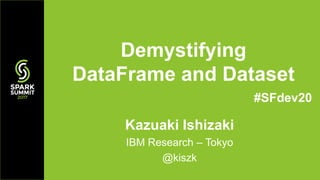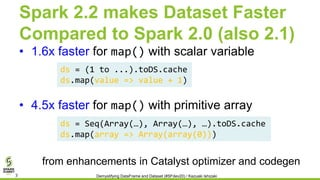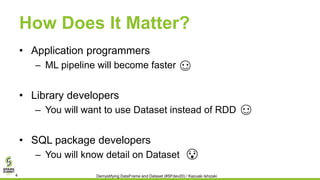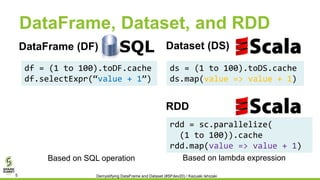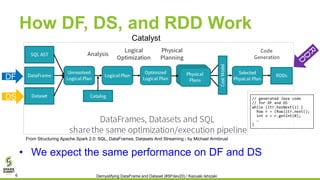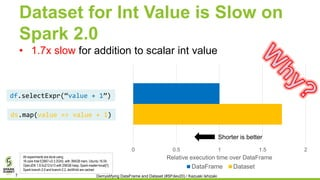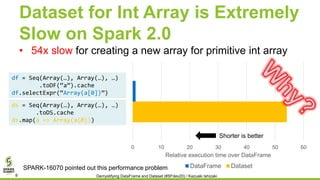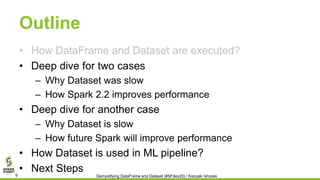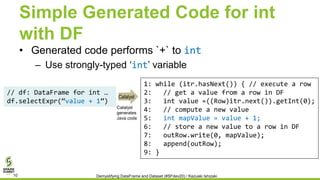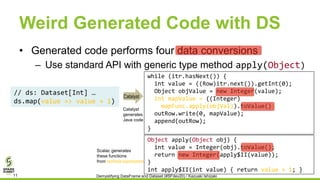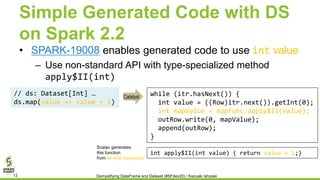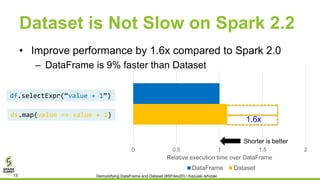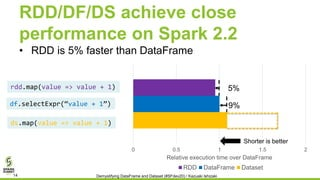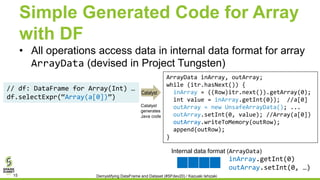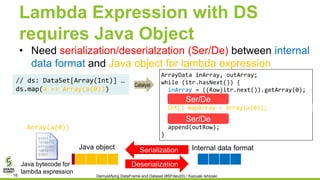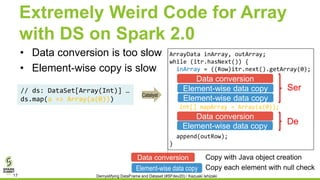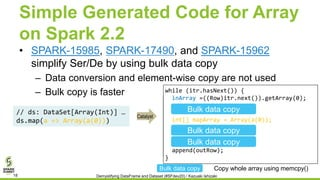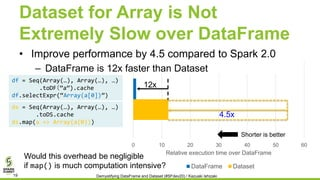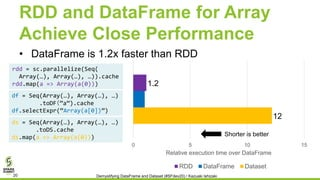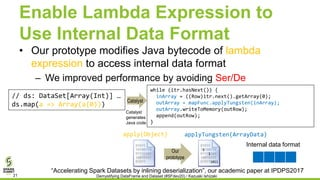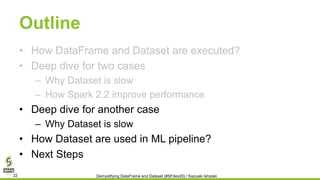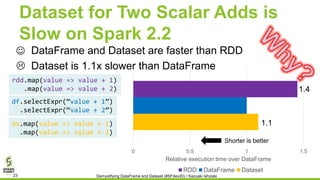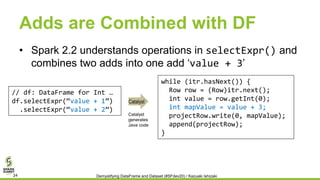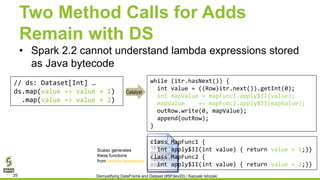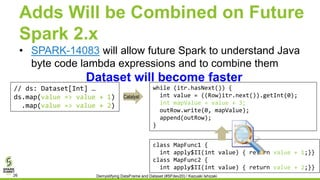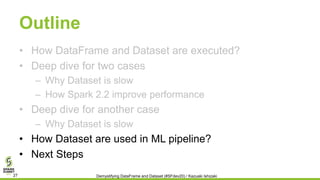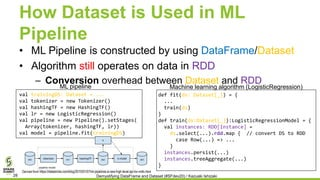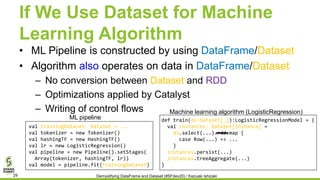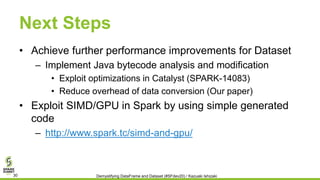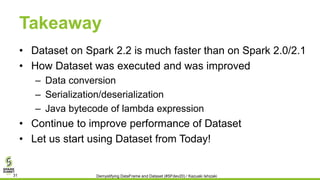1 of 32

### Demystifying DataFrame and Dataset with Kazuaki Ishizaki

1. Kazuaki Ishizaki IBM Research – Tokyo @kiszk Demystifying DataFrame and Dataset #SFdev20
2. About Me – Kazuaki Ishizaki • Researcher at IBM Research in compiler optimizations • Working for IBM Java virtual machine over 20 years – In particular, just-in-time compiler • Contributor to SQL package in Apache Spark • Committer of GPUEnabler – Apache Spark Plug-in to execute GPU code on Spark • https://github.com/IBMSparkGPU/GPUEnabler • Homepage: http://ibm.biz/ishizaki • Github: https://github.com/kiszk, Twitter: @kiszk Demystifying DataFrame and Dataset (#SFdev20) / Kazuaki Ishizaki2
3. Spark 2.2 makes Dataset Faster Compared to Spark 2.0 (also 2.1) • 1.6x faster for map() with scalar variable • 4.5x faster for map() with primitive array Demystifying DataFrame and Dataset (#SFdev20) / Kazuaki Ishizaki3 ds = (1 to ...).toDS.cache ds.map(value => value + 1) ds = Seq(Array(…), Array(…), …).toDS.cache ds.map(array => Array(array(0))) from enhancements in Catalyst optimizer and codegen
4. How Does It Matter? • Application programmers – ML pipeline will become faster • Library developers – You will want to use Dataset instead of RDD • SQL package developers – You will know detail on Dataset Demystifying DataFrame and Dataset (#SFdev20) / Kazuaki Ishizaki4
5. DataFrame, Dataset, and RDD df = (1 to 100).toDF.cache df.selectExpr(“value + 1”) ds = (1 to 100).toDS.cache ds.map(value => value + 1) SQLDataFrame (DF) Dataset (DS) Demystifying DataFrame and Dataset (#SFdev20) / Kazuaki Ishizaki5 RDD rdd = sc.parallelize( (1 to 100)).cache rdd.map(value => value + 1) Based on lambda expressionBased on SQL operation
6. How DF, DS, and RDD Work • We expect the same performance on DF and DS From Structuring Apache Spark 2.0: SQL, DataFrames, Datasets And Streaming - by Michael Armbrust DS DF Demystifying DataFrame and Dataset (#SFdev20) / Kazuaki Ishizaki6 Catalyst // generated Java code // for DF and DS while (itr.hasNext()) { Row r = (Row)itr.next(); int v = r.getInt(0); … }
7. Dataset for Int Value is Slow on Spark 2.0 • 1.7x slow for addition to scalar int value 0 0.5 1 1.5 2 Relative execution time over DataFrame DataFrame Dataset df.selectExpr(“value + 1”) ds.map(value => value + 1) All experiments are done using 16-core Intel E2667-v3 3.2GHz, with 384GB mem, Ubuntu 16.04, OpenJDK 1.8.0u212-b13 with 256GB heap, Spark master=local Spark branch-2.0 and branch-2.2, ds/df/rdd are cached Demystifying DataFrame and Dataset (#SFdev20) / Kazuaki Ishizaki7 Shorter is better
8. Dataset for Int Array is Extremely Slow on Spark 2.0 • 54x slow for creating a new array for primitive int array 0 10 20 30 40 50 60 Relative execution time over DataFrame DataFrame Dataset df = Seq(Array(…), Array(…), …) .toDF(“a”).cache df.selectExpr(“Array(a)”) ds = Seq(Array(…), Array(…), …) .toDS.cache ds.map(a => Array(a(0))) SPARK-16070 pointed out this performance problem Demystifying DataFrame and Dataset (#SFdev20) / Kazuaki Ishizaki8 Shorter is better
9. Outline • How DataFrame and Dataset are executed? • Deep dive for two cases – Why Dataset was slow – How Spark 2.2 improves performance • Deep dive for another case – Why Dataset is slow – How future Spark will improve performance • How Dataset is used in ML pipeline? • Next Steps Demystifying DataFrame and Dataset (#SFdev20) / Kazuaki Ishizaki9
10. Simple Generated Code for int with DF • Generated code performs `+` to int – Use strongly-typed ‘int’ variable 1: while (itr.hasNext()) { // execute a row 2: // get a value from a row in DF 3: int value =((Row)itr.next()).getInt(0); 4: // compute a new value 5: int mapValue = value + 1; 6: // store a new value to a row in DF 7: outRow.write(0, mapValue); 8: append(outRow); 9: } // df: DataFrame for int … df.selectExpr(“value + 1”) Demystifying DataFrame and Dataset (#SFdev20) / Kazuaki Ishizaki10 Catalyst generates Java code Catalyst
11. Weird Generated Code with DS • Generated code performs four data conversions – Use standard API with generic type method apply(Object) while (itr.hasNext()) { int value = ((Row)itr.next()).getInt(0); Object objValue = new Integer(value); int mapValue = ((Integer) mapFunc.apply(objVal)).toValue(); outRow.write(0, mapValue); append(outRow); } Object apply(Object obj) { int value = Integer(obj).toValue(); return new Integer(apply\$II(value)); } int apply\$II(int value) { return value + 1; } // ds: Dataset[Int] … ds.map(value => value + 1) Demystifying DataFrame and Dataset (#SFdev20) / Kazuaki Ishizaki11 Catalyst generates Java code Scalac generates these functions from lambda expression Catalyst
12. Simple Generated Code with DS on Spark 2.2 • SPARK-19008 enables generated code to use int value – Use non-standard API with type-specialized method apply\$II(int) while (itr.hasNext()) { int value = ((Row)itr.next()).getInt(0); int mapValue = mapFunc.apply\$II(value); outRow.write(0, mapValue); append(outRow); } int apply\$II(int value) { return value + 1;} // ds: Dataset[Int] … ds.map(value => value + 1) Demystifying DataFrame and Dataset (#SFdev20) / Kazuaki Ishizaki12 Catalyst Scalac generates this function from lambda expression
13. Dataset is Not Slow on Spark 2.2 0 0.5 1 1.5 2 Relative execution time over DataFrame DataFrame Dataset df.selectExpr(“value + 1”) ds.map(value => value + 1) Shorter is better Demystifying DataFrame and Dataset (#SFdev20) / Kazuaki Ishizaki13 1.6x • Improve performance by 1.6x compared to Spark 2.0 – DataFrame is 9% faster than Dataset
14. RDD/DF/DS achieve close performance on Spark 2.2 • RDD is 5% faster than DataFrame 0 0.5 1 1.5 2 Relative execution time over DataFrame RDD DataFrame Dataset df.selectExpr(“value + 1”) ds.map(value => value + 1) rdd.map(value => value + 1) Demystifying DataFrame and Dataset (#SFdev20) / Kazuaki Ishizaki14 9% 5% Shorter is better
15. Simple Generated Code for Array with DF • All operations access data in internal data format for array ArrayData (devised in Project Tungsten) // df: DataFrame for Array(Int) … df.selectExpr(“Array(a)”) ArrayData inArray, outArray; while (itr.hasNext()) { inArray = ((Row)itr.next()).getArray(0); int value = inArray.getInt(0)); //a outArray = new UnsafeArrayData(); ... outArray.setInt(0, value); //Array(a) outArray.writeToMemory(outRow); append(outRow); } Demystifying DataFrame and Dataset (#SFdev20) / Kazuaki Ishizaki15 Catalyst generates Java code Internal data format (ArrayData) inArray.getInt(0) outArray.setInt(0, …) Catalyst
16. Lambda Expression with DS requires Java Object • Need serialization/deserialzation (Ser/De) between internal data format and Java object for lambda expression // ds: DataSet[Array(Int)] … ds.map(a => Array(a(0))) ArrayData inArray, outArray; while (itr.hasNext()) { inArray = ((Row)itr.next()).getArray(0); append(outRow); } Internal data formatJava object Ser/De Array(a(0)) Demystifying DataFrame and Dataset (#SFdev20) / Kazuaki Ishizaki16 int[] mapArray = Array(a(0)); Java bytecode for lambda expression Catalyst Deserialization Serialization Ser/De
17. ArrayData inArray, outArray; while (itr.hasNext()) { inArray = ((Row)itr.next().getArray(0); Object[] tmp = new Object[inArray.numElements()]; for (int i = 0; i < tmp.length; i ++) { tmp[i] = (inArray.isNullAt(i)) ? Null : inArray.getInt(i); } ArrayData array = new GenericIntArrayData(tmpArray); int[] javaArray = array.toIntArray(); int[] mapArray = (int[])map_func.apply(javaArray); outArray = new GenericArrayData(mapArray); for (int i = 0; i < outArray.numElements(); i++) { if (outArray.isNullAt(i)) { arrayWriter.setNullInt(i); } else { arrayWriter.write(i, outArray.getInt(i)); } } append(outRow); } ArrayData inArray, outArray; while (itr.hasNext()) { inArray = ((Row)itr.next().getArray(0); append(outRow); } Extremely Weird Code for Array with DS on Spark 2.0 • Data conversion is too slow • Element-wise copy is slow // ds: DataSet[Array(Int)] … ds.map(a => Array(a(0))) Data conversion Element-wise data copy Element-wise data copy Demystifying DataFrame and Dataset (#SFdev20) / Kazuaki Ishizaki17 Data conversion Copy with Java object creation Element-wise data copy Copy each element with null check int[] mapArray = Array(a(0)); Catalyst Data conversion Element-wise data copy Ser De
18. Simple Generated Code for Array on Spark 2.2 • SPARK-15985, SPARK-17490, and SPARK-15962 simplify Ser/De by using bulk data copy – Data conversion and element-wise copy are not used – Bulk copy is faster // ds: DataSet[Array(Int)] … ds.map(a => Array(a(0))) while (itr.hasNext()) { inArray =((Row)itr.next()).getArray(0); int[] javaArray = inArray.toIntArray(); int[] mapArray = (int[])mapFunc.apply(javaArray); outArray = UnsafeArrayData .fromPrimitiveArray(mapArray); outArray.writeToMemory(outRow); append(outRow); } Demystifying DataFrame and Dataset (#SFdev20) / Kazuaki Ishizaki18 Bulk data copy Copy whole array using memcpy() Catalyst while (itr.hasNext()) { inArray =((Row)itr.next()).getArray(0); int[]mapArray=(int[])map.apply(javaArray); append(outRow); } Bulk data copy int[] mapArray = Array(a(0)); Bulk data copy Bulk data copy
19. 0 10 20 30 40 50 60 Relative execution time over DataFrame DataFrame Dataset Dataset for Array is Not Extremely Slow over DataFrame • Improve performance by 4.5 compared to Spark 2.0 – DataFrame is 12x faster than Dataset ds = Seq(Array(…), Array(…), …) .toDS.cache ds.map(a => Array(a(0))) Demystifying DataFrame and Dataset (#SFdev20) / Kazuaki Ishizaki19 4.5x df = Seq(Array(…), Array(…), …) .toDF（”a”).cache df.selectExpr(“Array(a)”) Would this overhead be negligible if map() is much computation intensive? 12x Shorter is better
20. RDD and DataFrame for Array Achieve Close Performance • DataFrame is 1.2x faster than RDD 0 5 10 15 Relative execution time over DataFrame RDD DataFrame Dataset ds = Seq(Array(…), Array(…), …) .toDS.cache ds.map(a => Array(a(0))) Demystifying DataFrame and Dataset (#SFdev20) / Kazuaki Ishizaki20 df = Seq(Array(…), Array(…), …) .toDF（”a”).cache df.selectExpr(“Array(a)”) rdd = sc.parallelize(Seq( Array(…), Array(…), …)).cache rdd.map(a => Array(a(0))) 1.2 12 Shorter is better
21. Enable Lambda Expression to Use Internal Data Format • Our prototype modifies Java bytecode of lambda expression to access internal data format – We improved performance by avoiding Ser/De // ds: DataSet[Array(Int)] … ds.map(a => Array(a(0))) while (itr.hasNext()) { inArray = ((Row)itr.next().getArray(0); outArray = mapFunc.applyTungsten(inArray); outArray.writeToMemory(outRow); append(outRow); } Internal data format “Accelerating Spark Datasets by inlining deserialization”, our academic paper at IPDPS2017 1 1 apply(Object) 1011 applyTungsten(ArrayData) Demystifying DataFrame and Dataset (#SFdev20) / Kazuaki Ishizaki21 Catalyst generates Java code Catalyst Our prototype
22. Outline • How DataFrame and Dataset are executed? • Deep dive for two cases – Why Dataset is slow – How Spark 2.2 improve performance • Deep dive for another case – Why Dataset is slow • How Dataset are used in ML pipeline? • Next Steps Demystifying DataFrame and Dataset (#SFdev20) / Kazuaki Ishizaki22
23. 0 0.5 1 1.5 Relative execution time over DataFrame RDD DataFrame Dataset Dataset for Two Scalar Adds is Slow on Spark 2.2 • DataFrame and Dataset are faster than RDD • Dataset is 1.1x slower than DataFrame df.selectExpr(“value + 1”) .selectExpr(“value + 2”) ds.map(value => value + 1) .map(value => value + 2) Demystifying DataFrame and Dataset (#SFdev20) / Kazuaki Ishizaki23 rdd.map(value => value + 1) .map(value => value + 2) Shorter is better ☺  1.1 1.4
24. Adds are Combined with DF • Spark 2.2 understands operations in selectExpr() and combines two adds into one add ‘value + 3’ while (itr.hasNext()) { Row row = (Row)itr.next(); int value = row.getInt(0); int mapValue = value + 3; projectRow.write(0, mapValue); append(projectRow); } // df: DataFrame for Int … df.selectExpr(“value + 1”) .selectExpr(“value + 2”) Demystifying DataFrame and Dataset (#SFdev20) / Kazuaki Ishizaki24 Catalyst Catalyst generates Java code
25. Two Method Calls for Adds Remain with DS • Spark 2.2 cannot understand lambda expressions stored as Java bytecode while (itr.hasNext()) { int value = ((Row)itr.next()).getInt(0); int mapValue = mapFunc1.apply\$II(value); mapValue += mapFunc2.apply\$II(mapValue); outRow.write(0, mapValue); append(outRow); } // ds: Dataset[Int] … ds.map(value => value + 1) .map(value => value + 2) class MapFunc1 { int apply\$II(int value) { return value + 1;}} class MapFunc2 { int apply\$II(int value) { return value + 2;}} Demystifying DataFrame and Dataset (#SFdev20) / Kazuaki Ishizaki25 Catalyst Scalac generates these functions from lambda expression
26. class MapFunc1 { int apply\$II(int value) { return value + 1;}} class MapFunc2 { int apply\$II(int value) { return value + 2;}} Adds Will be Combined on Future Spark 2.x • SPARK-14083 will allow future Spark to understand Java byte code lambda expressions and to combine them while (itr.hasNext()) { int value = ((Row)itr.next()).getInt(0); int mapValue = value + 3; outRow.write(0, mapValue); append(outRow); } // ds: Dataset[Int] … ds.map(value => value + 1) .map(value => value + 2) Dataset will become faster Demystifying DataFrame and Dataset (#SFdev20) / Kazuaki Ishizaki26 Catalyst
27. Outline • How DataFrame and Dataset are executed? • Deep dive for two cases – Why Dataset is slow – How Spark 2.2 improve performance • Deep dive for another case – Why Dataset is slow • How Dataset are used in ML pipeline? • Next Steps Demystifying DataFrame and Dataset (#SFdev20) / Kazuaki Ishizaki27
28. How Dataset is Used in ML Pipeline • ML Pipeline is constructed by using DataFrame/Dataset • Algorithm still operates on data in RDD – Conversion overhead between Dataset and RDD val trainingDS: Dataset = ... val tokenizer = new Tokenizer() val hashingTF = new HashingTF() val lr = new LogisticRegression() val pipeline = new Pipeline().setStages( Array(tokenizer, hashingTF, lr)) val model = pipeline.fit(trainingDS) def fit(ds: Dataset[_]) = { ... train(ds) } def train(ds:Dataset[_]):LogisticRegressionModel = { val instances: RDD[Instance] = ds.select(...).rdd.map { // convert DS to RDD case Row(...) => ... } instances.persist(...) instances.treeAggregate(...) } ML pipeline Machine learning algorithm (LogisticRegression) Derived from https://databricks.com/blog/2015/01/07/ml-pipelines-a-new-high-level-api-for-mllib.html Demystifying DataFrame and Dataset (#SFdev20) / Kazuaki Ishizaki28
29. If We Use Dataset for Machine Learning Algorithm • ML Pipeline is constructed by using DataFrame/Dataset • Algorithm also operates on data in DataFrame/Dataset – No conversion between Dataset and RDD – Optimizations applied by Catalyst – Writing of control flows val trainingDataset: Dataset = ... val tokenizer = new Tokenizer() val hashingTF = new HashingTF() val lr = new LogisticRegression() val pipeline = new Pipeline().setStages( Array(tokenizer, hashingTF, lr)) val model = pipeline.fit(trainingDataset) def train(ds:Dataset[_]):LogisticRegressionModel = { val instances: Dataset[Instance] = ds.select(...).rdd.map { case Row(...) => ... } instances.persist(...) instances.treeAggregate(...) } ML pipeline Demystifying DataFrame and Dataset (#SFdev20) / Kazuaki Ishizaki29 Machine learning algorithm (LogisticRegression)
30. Next Steps • Achieve further performance improvements for Dataset – Implement Java bytecode analysis and modification • Exploit optimizations in Catalyst (SPARK-14083) • Reduce overhead of data conversion (Our paper) • Exploit SIMD/GPU in Spark by using simple generated code – http://www.spark.tc/simd-and-gpu/ Demystifying DataFrame and Dataset (#SFdev20) / Kazuaki Ishizaki30
31. Takeaway • Dataset on Spark 2.2 is much faster than on Spark 2.0/2.1 • How Dataset was executed and was improved – Data conversion – Serialization/deserialization – Java bytecode of lambda expression • Continue to improve performance of Dataset • Let us start using Dataset from Today! Demystifying DataFrame and Dataset (#SFdev20) / Kazuaki Ishizaki31
32. Thank you @kiszk on twitter @kiszk on github http://ibm.biz/ishizaki • @sameeragarwal, @hvanhovell, @gatorsmile, @cloud-fan, @ueshin, @davies, @rxin, @joshrosen, @maropu, @viirya • Spark Technology Center